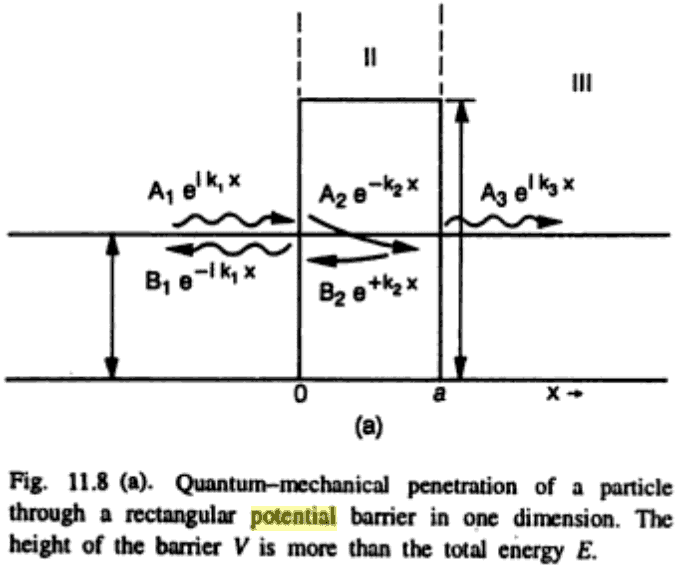# Particle waves through a potential barrier

Hi all,

I came across this figure in a textbook. Simple stuff, but can get tricky:I don't understand why the sign convention flips upon entering the barrier (region II), but I guess the book is correct and that I should just take it as a fact.

If anybody has a reasonable thought to add so I'm making sense out of this rather than just accepting it, please let me know.

Thanks!

blue_leaf77
Homework Helper
Physical intuition, a barrier is supposed to prevent a particle from passing through, therefore we must expect the probability to find the particle beyond the barrier is lower than it is before.

What do you mean by sign convention? The solution to the schrodinger equation for a particle in a region where the potential is larger than the energy is a sum of a decaying and growing exponentials.

jtbell
Mentor
You're free to swap the signs on the exponents in the A2 and B2 terms when setting up the solution, but then the values of A2 and B2 will also get swapped when you actually work out the solution.

Last edited:
Hey thanks for the explanation, jtbell. That makes sense now.